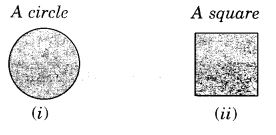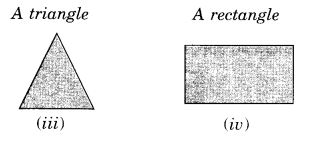NCERT Solutions for Class 7 Maths Chapter 15 Visualising Solid Shapes Ex 15.4 are part of NCERT Solutions for Class 7 Maths. Here we have given NCERT Solutions for Class 7 Maths Chapter 15 Visualising Solid Shapes Ex 15.4.

 Board CBSE Textbook NCERT Class Class 7 Subject Maths Chapter Chapter 15 Chapter Name Visualising Solid Shapes Exercise Ex 15.4 Number of Questions Solved 3 Category NCERT Solutions

## NCERT Solutions for Class 7 Maths Chapter 15 Visualising Solid Shapes Ex 15.4

Question 1.
A bulb is kept burning just right above the following solids. Name the shape of the shadows obtained in each case. Attempt to give a rough sketch of the shadow. (You may try to experiment first and then answer these questions):Solution:

1. a circle.
2. a rectangle.
3. a rectangle.

Question 2.
Here are the shadows of some 3-D objects, when seen under the lamp of an overhead projector. Identify the solid(s) that match each shadow. (There may be multiple answers for these!)Solution:

1. → Sphere, cylinder
2. → Cube, cuboid (of the square end)
3. → Cone, the prism of the triangular base
4. → Cylinder, the prism of the square base

Question 3.
Examine if the following are true statements:

1. The cube can cast a shadow in the shape of a rectangle.
2. The cube can cast a shadow in the shape of a hexagon.

Solution:

1. True, the cube can cast a shadow in the shape of a rectangle.
2. False, the cube can not cast a shadow in the shape of a hexagon.

We hope the NCERT Solutions for Class 7 Maths Chapter 15 Visualising Solid Shapes Ex 15.4 helps you. If you have any query regarding NCERT Solutions for Class 7 Maths Chapter 15 Visualising Solid Shapes Ex 15.4, drop a comment below and we will get back to you at the earliest.